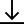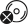# Mental Computation - Using Natural Maths Strategies - Lower Primary

\$24.95

Instant DownloadCD not includedMental computation is the process of drawing on known strategies to solve an arithmetical problem. As a process it differs from mental arithmetic where the emphasis is on fast recall of known number facts, rather than having a way of working out what the answer might be. For example, to find 7+8+3, a strategic approach would be to change the order of the addition to 7+3+8 because you know that 7+3 makes 10, a rainbow fact, and that adding 8 to 10 is easy because 10 is a friendly number.

This downloadable PDF resource provides activities that support mental computation by exploring Natural Maths Strategies posters. The posters present the early addition and subtraction strategies and lay the foundation for the understanding and later recalling of addition and subtraction facts. Knowledge of these strategies ensures that, in a situation where memory fails or the numbers are too large, the individual has the confidence to use mental computation to derive the answer.

This resource includes:

• 18 Natural Maths Strategies posters in colour in A4
• 16 resource cards

Topics include:

• Subitise
• Count on
• Double
• Rainbow Facts
• Turnarounds
• Near Double
• Secret Code
• And many more…

SKU:
9781925269994
Year:
Primary - Lower
Subject:
Mathematics
Series:
Other